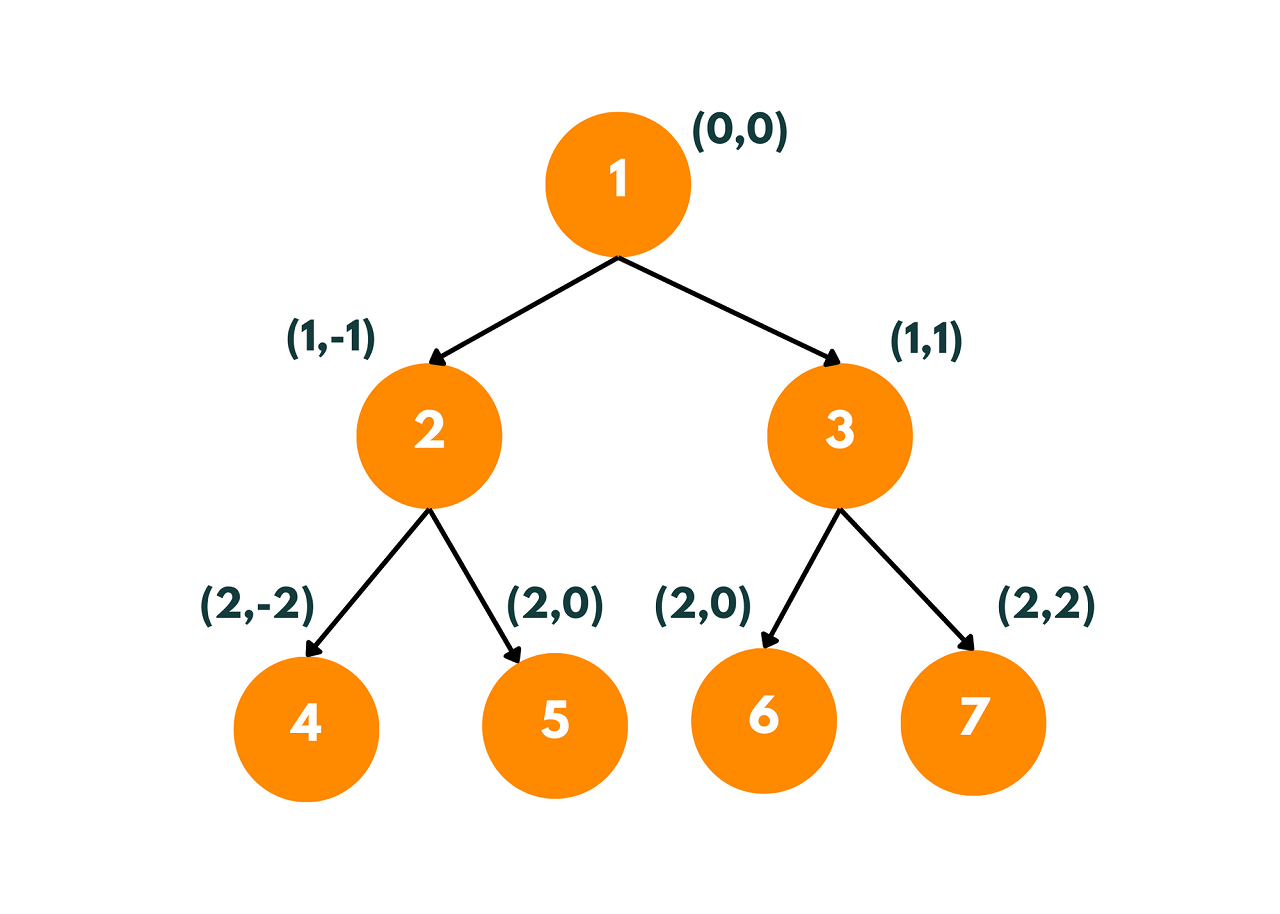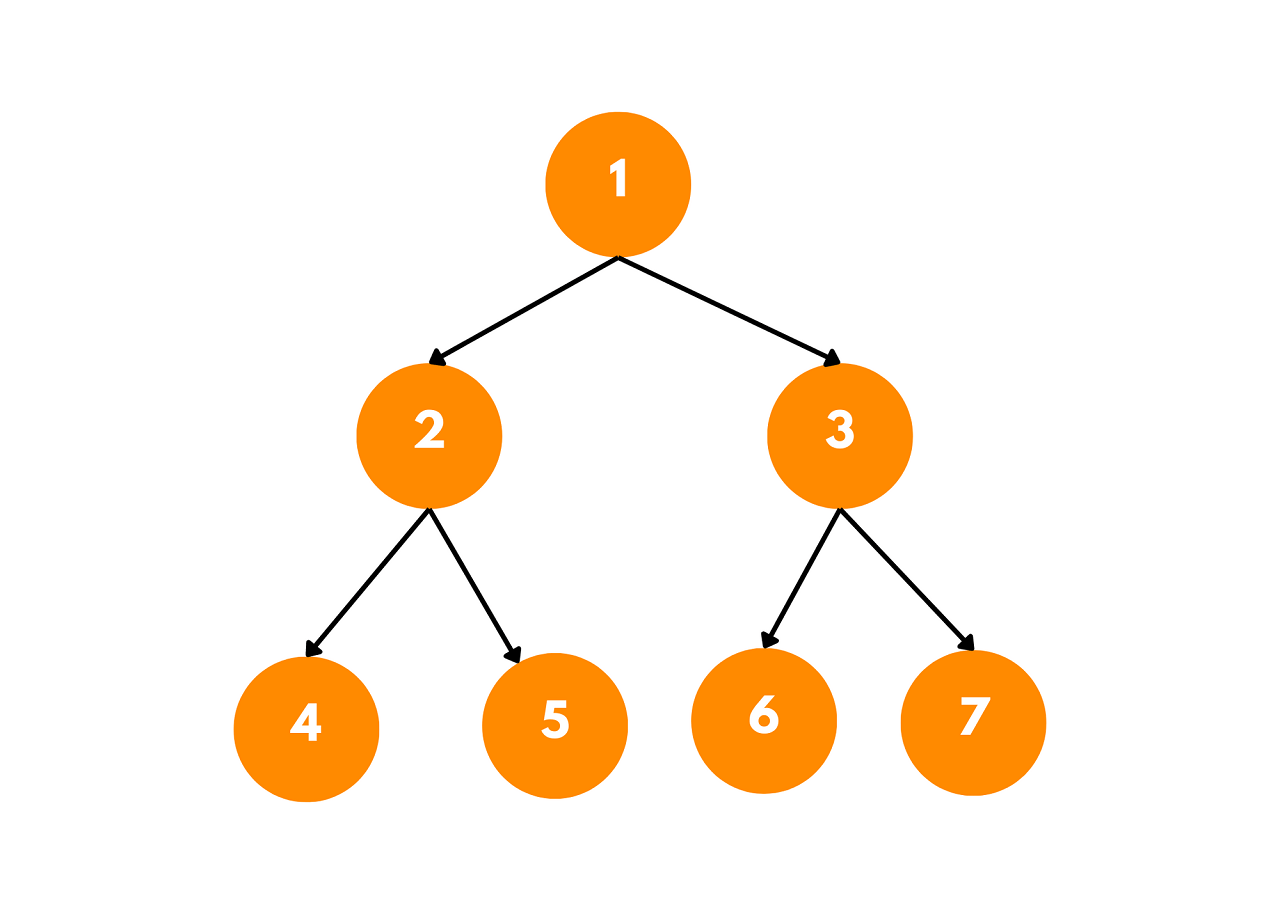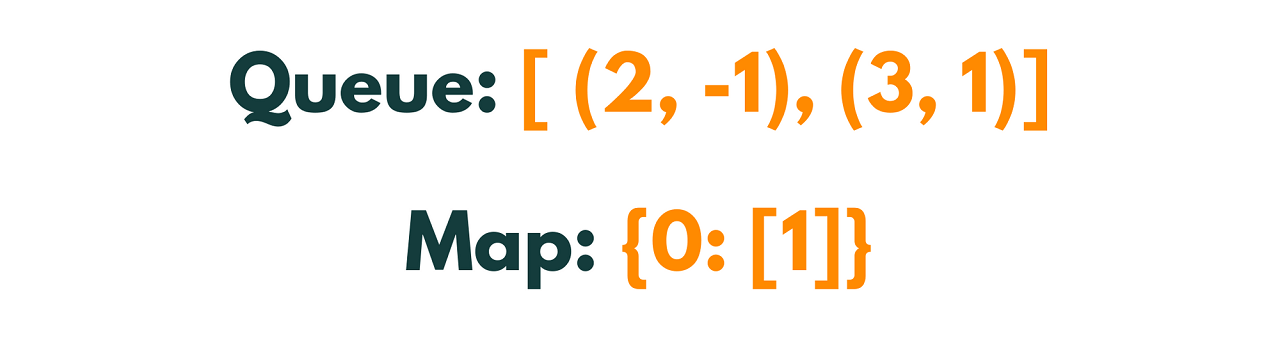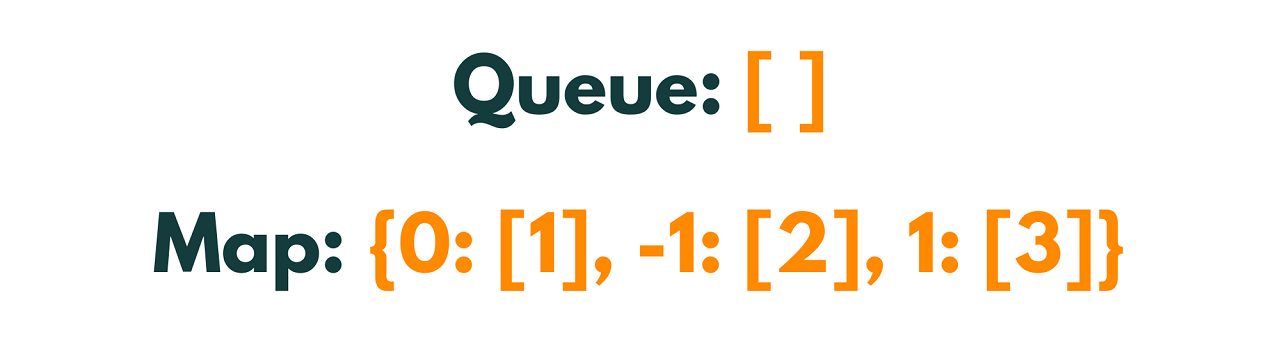# Vertical Order Traversal of Binary Tree (with code)

Binary trees are fundamental data structures in computer science that serve as the building blocks for many algorithms. Traversing a binary tree is a common operation, and various traversal techniques exist to explore the nodes of a tree in different orders. One such traversal is the Vertical Order Traversal of a Binary Tree, which we will learn in this article, with implementation in Python.

## Vertical Order Traversal of Binary Tree

Vertical Order Traversal is a method of traversing a binary tree that arranges the nodes based on their vertical positions. Each node in the tree is assigned a horizontal distance value, with the root node having a distance of 0. Nodes to the left of the root have negative distance values, while nodes to the right have positive distance values.The vertical order of the above tree is [4, 2, 1, 5, 6, 3, 8, 7]

The traversal follows a top-to-bottom approach, scanning the tree from left to right. When two nodes have the same horizontal distance, the node that appears higher in the tree is visited first. This process continues until all the nodes have been traversed, resulting in a vertical order sequence of the tree's elements.

### Implementing Vertical Order Traversal

To implement Vertical Order Traversal in a binary tree, we can employ a modified form of level order traversal using a queue and a map. The steps involved in the process are as follows:

1. Create an empty queue to hold nodes, along with an empty map to store nodes based on their horizontal distances.

2. Enqueue the root node into the queue along with its horizontal distance, which is initially set to 0.

3. Perform a level order traversal by dequeuing nodes from the queue one by one.

4. For each dequeued node, store its value in the map based on its horizontal distance. If the horizontal distance already exists in the map, append the node's value to the corresponding list.

5. If the dequeued node has a left child, enqueue it into the queue with its horizontal distance decremented by 1.

6. If the dequeued node has a right child, enqueue it into the queue with its horizontal distance incremented by 1.

7. Repeat steps 3-6 until the queue becomes empty.

8. After traversing all the nodes, the map will contain the elements arranged vertically based on their horizontal distances.

9. Iterate through the map and print the values from left to right for each horizontal distance.

### Example of Vertical Order Traversal

Let's consider the following binary tree as an example:To perform the Vertical Order Traversal on this binary tree, we'll go through the step-by-step process explained earlier.

Step 1: Enqueue the root node with a horizontal distance of 0.Step 2: Dequeue the node (1, 0) and store its value (1) in the map at distance 0.Step 3: Check for left and right children of the dequeued node and enqueue them with their respective horizontal distances.Step 4: Dequeue the node (2, -1) and store its value (2) in the map at a distance of -1.Step 5: Dequeue the node (3, 1) and store its value (3) in the map at distance 1.Step 6: Check for left and right children of the dequeued node (2, -1). Since it has no children, the queue remains unchanged.

Step 7: Check for left and right children of the dequeued node (3, 1). Since it has no children, the queue remains unchanged.

Step 8: The queue is now empty, and we have traversed all the nodes.

Step 9: Iterate through the map and print the values from left to right for each horizontal distance.

The vertical order traversal of the given binary tree results in the sequence [4, 2, 1, 5, 6, 3, 7]. This sequence represents the nodes arranged based on their vertical positions, with nodes having a lower horizontal distance appearing first.

### Python Code for Vertical Order Traversal

Here's an example of Python code for performing a vertical order traversal on a binary tree:

```from collections import defaultdict, deque

# Definition of a binary tree node
class TreeNode:
def __init__(self, val):
self.val = val
self.left = None
self.right = None

def vertical_order_traversal(root):
# Base case: if the tree is empty
if not root:
return []

# Create a dictionary to store nodes based on their horizontal distances
vertical_order = defaultdict(list)

# Create a queue for level order traversal
queue = deque()
queue.append((root, 0))  # Pairing each node with its horizontal distance

# Perform level order traversal
while queue:
node, distance = queue.popleft()

# Add the current node to the dictionary
vertical_order[distance].append(node.val)

# Enqueue the left child with the updated horizontal distance
if node.left:
queue.append((node.left, distance - 1))

# Enqueue the right child with the updated horizontal distance
if node.right:
queue.append((node.right, distance + 1))

# Sort the keys (horizontal distances) in ascending order
sorted_distances = sorted(vertical_order.keys())

# Retrieve the nodes in vertical order
result = []
for distance in sorted_distances:
result.extend(vertical_order[distance])

return result

# Example usage:
# Create the binary tree
root = TreeNode(1)
root.left = TreeNode(2)
root.right = TreeNode(3)
root.left.left = TreeNode(4)
root.left.right = TreeNode(5)
root.right.left = TreeNode(6)
root.right.right = TreeNode(7)

# Perform vertical order traversal
result = vertical_order_traversal(root)

# Print the result
print(result)```

Output:

```[4, 2, 1, 5, 6, 3, 7]
```

This code uses a 'defaultdict' from the collections module to store the nodes based on their horizontal distances. It performs a level order traversal in Python using a queue, enqueuing the nodes along with their updated horizontal distances. Finally, it sorts the keys (horizontal distances) and retrieves the nodes in the desired vertical order.

Please note that the code assumes the binary tree is represented using the TreeNode class, which includes a val attribute for the node's value, as well as left and right attributes for the left and right children, respectively. You can modify the code as per your specific binary tree implementation if needed.

### Time and Space Complexity

The time complexity of the code is O(N), where N is the number of nodes in the binary tree. This is because we perform a level order traversal, visiting each node once. In the worst case, we need to visit all nodes in the tree to complete the traversal. The space complexity of the code is O(N).

Also, learn how to invert a binary tree as well.

## Conclusion

Vertical Order Traversal provides valuable insights into the hierarchical structure of a binary tree. It allows us to visualize the tree nodes based on their vertical positions, which can be useful in various scenarios, including Printing a binary tree in a column-wise fashion or grouping nodes with the same horizontal distance together.

### FavTutor - 24x7 Live Coding Help from Expert Tutors!